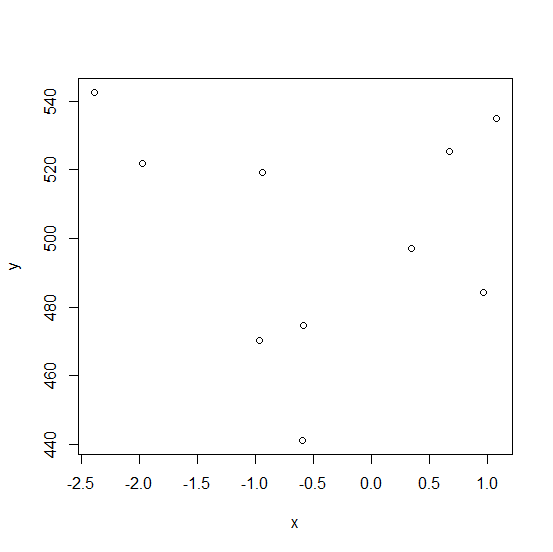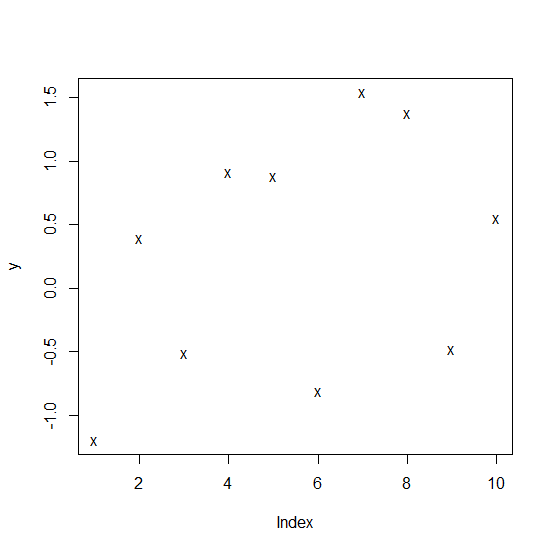# How to find the size of the plotting window in R?

The plotting window size can be found by using dev.size function and we can pass in for inches and cm for centimeters. For example, if we create a plot then we can use dev.size("in") to find the plot size in inches and dev.size("cm") to find the size in centimeters.

## Example

Consider the below vectors and create a point chart between those vectors −

Live Demo

x<-rnorm(10)
y<-rnorm(10,500,35)
plot(x,y)

## OutputNow finding the plotting window size −

## Example

dev.size("in") # in inches

## Output

 5.760417 5.750000

## Example

dev.size("cm") # in centimeters


## Output

 14.63146 14.60500

This will change if we drag the plotting window to right, left, upper or down side as shown below (dragging the plotting window to left) −Now again finding the size −

## Example

dev.size("in")

## Output

 6.614583 5.750000

## Example

dev.size("cm")


## Output

 16.80104 14.60500

This will not change until and unless we do not increase the plotting window manually.